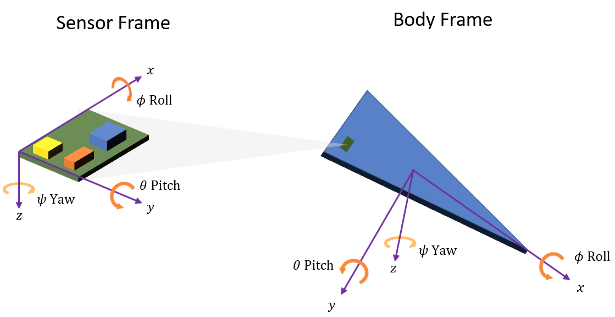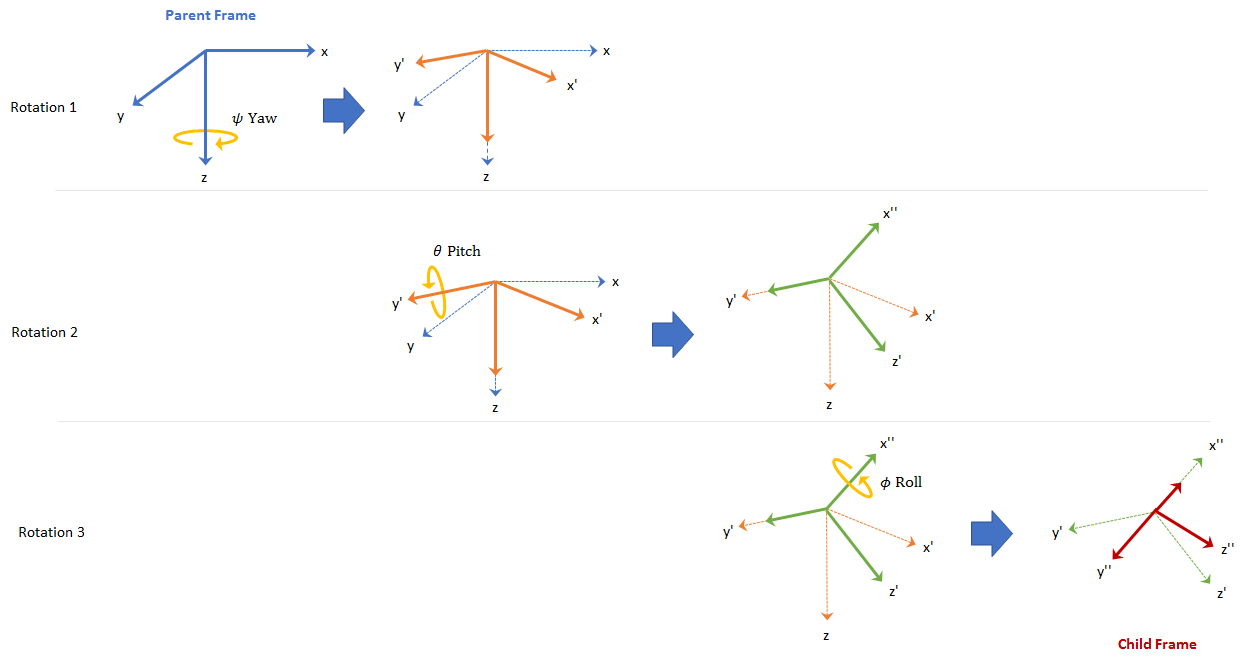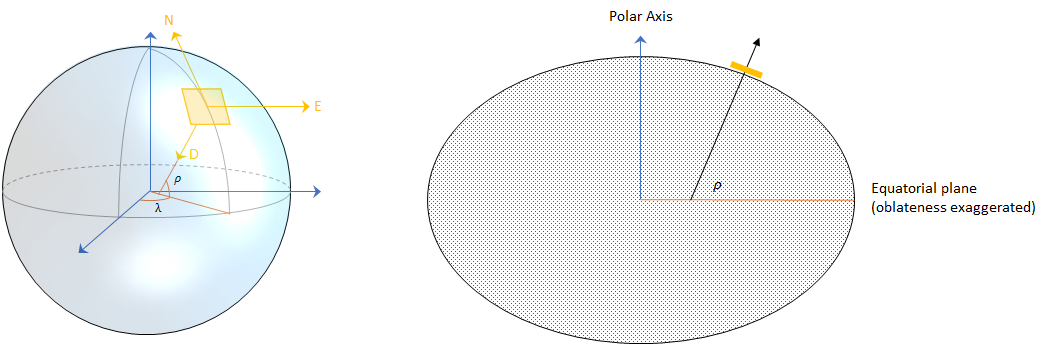Documentation

## Orientation, Position, and Coordinate Systems

The Sensor Fusion and Tracking Toolbox™ enables you to track orientation, position, pose, and trajectory of a platform. A platform refers generally to any object you want to track.

### Orientation

Orientation is defined by angular displacement. Orientation can be described in terms of point or frame rotation. In point rotation, the coordinate system is static and the point moves. In frame rotation, the point is static and the coordinate system moves. For a given axis and angle of rotation, point rotation and frame rotation define equivalent angular displacement but in opposite directions.

Sensor Fusion and Tracking Toolbox defaults to frame rotation.Orientation is defined as the frame rotation that takes the parent frame to the child frame. The choice of parent frame depends on the problem space. For example, manipulating sensor frames is necessary to align various axes of independent sensors. Tracking the body frame is often used for stabilization tasks. The ground reference frame is useful for tracking multiple independent platforms and locating platforms in an absolute sense.

Sensor Fusion and Tracking Toolbox primarily supports the NED (north-east-down) coordinate frame. You can also use the ENU (east-north-up) coordinate frame in many features.### Frame Rotation

To relate one orientation to another you must rotate a frame. The table summarizes the rotation conventions that Sensor Fusion and Tracking Toolbox uses. A three-axis coordinate is always specified in order [x,y,z].

VariableEuler AngleSymbolOutput Interval (Degrees)
zYawψ

−180 ≤ ψ < 180

yPitchθ

−90 ≤ θ ≤ 90

xRollϕ

−180 ≤ ϕ < 180

A positive rotation angle corresponds to a clockwise rotation about an axis when viewing from the origin along the positive direction of the axis. The right-hand convention is equivalent, where positive rotation is indicated by the direction in which the fingers on your right hand curl when your thumb is pointing in the direction of the axis of rotation.

To define three-dimensional frame rotation, you must rotate sequentially about the axes. Sensor Fusion and Tracking Toolbox uses intrinsic (carried frame) rotation, in which, after each rotation, the axis is updated before the next rotation. For example, to rotate an axis using the ZYX convention:

1. Rotate the parent frame about the z-axis to yield a new set of axes, (x',y',z), where the x- and y-axes have changed to x'- and y'-axes and the z-axis remains unchanged.

`$\left[\begin{array}{c}{x}^{\text{'}}\\ {y}^{\text{'}}\\ z\end{array}\right]={\text{R}}_{z}\left(\psi \right)\left[\begin{array}{c}x\\ y\\ z\end{array}\right]$`
2. Rotate the new set of axes about the y'-axis, yielding another new set of axes, (x'',y',z').

`$\left[\begin{array}{c}{x}^{\text{'}\text{'}}\\ {y}^{\text{'}}\\ {z}^{\text{'}}\end{array}\right]={\text{R}}_{y}\left(\theta \right)\left[\begin{array}{c}{x}^{\text{'}}\\ {y}^{\text{'}}\\ z\end{array}\right]$`
3. Rotate this new set of axes about the x''-axis, arriving at the desired child frame, (x'',y'',z'').

`$\left[\begin{array}{c}{x}^{\text{'}\text{'}}\\ {y}^{\text{'}\text{'}}\\ {z}^{\text{'}\text{'}}\end{array}\right]={\text{R}}_{x}\left(\varphi \right)\left[\begin{array}{c}{x}^{\text{'}\text{'}}\\ {y}^{\text{'}}\\ {z}^{\text{'}}\end{array}\right]$`This sequence of rotations follows the convention outlined in . The rotation matrix required to convert a vector in the parent frame to a vector in the child frame for a given yaw, pitch, and roll is computed as:

`$\text{R}\left(\psi ,\theta ,\varphi \right)={\text{R}}_{x}\left(\psi \right){\text{R}}_{y}\left(\theta \right){\text{R}}_{z}\left(\varphi \right)=\left[\begin{array}{ccc}\mathrm{cos}\psi \mathrm{cos}\theta & \mathrm{sin}\psi \mathrm{cos}\theta & -\mathrm{sin}\theta \\ \mathrm{cos}\psi \mathrm{sin}\theta \mathrm{sin}\varphi -\mathrm{sin}\psi \mathrm{cos}\varphi \text{ }& \mathrm{sin}\psi \mathrm{sin}\theta \mathrm{sin}\varphi +\mathrm{cos}\psi \mathrm{cos}\varphi \text{ }& \mathrm{cos}\theta \mathrm{sin}\varphi \\ \mathrm{cos}\psi \mathrm{sin}\theta \mathrm{cos}\varphi +\mathrm{sin}\psi \mathrm{cos}\varphi \text{ }& \mathrm{sin}\psi \mathrm{sin}\theta \mathrm{sin}\varphi +\mathrm{cos}\psi \mathrm{cos}\varphi \text{ }& \mathrm{cos}\theta \mathrm{cos}\varphi \end{array}\right]$`

For features that support frame-based processing, Sensor Fusion and Tracking Toolbox provides coordinates as an N-by-3 matrix, where N is the number of samples in time and the three columns correspond to the x-, y-, and z-axes. The following calculation rotates a parent frame to a child frame:

`${a}_{\text{child}}={\left(\text{R}\left(\psi ,\theta ,\varphi \right)×{\left({a}_{\text{parent}}\right)}^{\text{T}}\right)}^{\text{T}}$`

Sensor Fusion and Tracking Toolbox enables efficient orientation computation using the `quaternion` data type. To create a rotation matrix using quaternions, use the `rotmat` function.

```% Euler angles defining orientation of local axes yaw = 20; pitch = 5; roll = 10; % Create orientation matrix from Euler angles using quaternion class q = quaternion([yaw pitch roll],'eulerd','zyx','frame'); myRotationMatrix = rotmat(q,'frame'); ```
See Orientation, Position, and Coordinate Systems for more information on using quaternions in Sensor Fusion and Tracking Toolbox.

### Position

Position is defined as the translational distance from a parent frame origin to a child frame origin. For example, take the local NED coordinate system as the parent frame. In the NED coordinate system:

• The origin is arbitrarily fixed to a point on the surface of the Earth. This makes the NED coordinate system local.

• The x-axis points toward the ellipsoid north.

• The y-axis points toward the ellipsoid east.

• The z-axis points downward along the ellipsoid normal (geodetic latitude, ρ).#### Azimuth and Elevation

Given a vector in R3:

• Azimuth is defined as the angle from the x-axis to the orthogonal projection of the vector onto the xy-plane. The angle is positive going from the x-axis toward the y-axis. Azimuth is given in degrees in the range [−180, 180).

• Elevation is defined as the angle from the projection onto the xy-plane to the vector. The angle is positive going from the xy-plane to the z-axis. Elevation is given in degrees in the range [−90, 90].### Pose

To specify an object in 3-D space fully, you can combine position and orientation. Pose is defined as the combination of position and orientation. Sensor Fusion and Tracking Toolbox uses the following conventions when describing pose.

Property/FieldDescriptionUnitsCoordinate Frame
PositionCurrent position of platform in scenariomNED or ENU
VelocityCurrent velocity of platform in scenariom/sNED or ENU
AccelerationCurrent acceleration of platform in scenariom/s2NED or ENU
OrientationCurrent orientation of platform in scenariounit quaternion / orientation matrixN/A
Angular velocityCurrent angular velocity of platform in scenariorad/sNED or ENU

### Trajectory

Trajectory defines how pose changes over time. To generate ground-truth trajectories in Sensor Fusion and Tracking Toolbox, use `kinematicTrajectory` or `waypointTrajectory`. To simulate tracking multiple platforms, use `trackingScenario`.